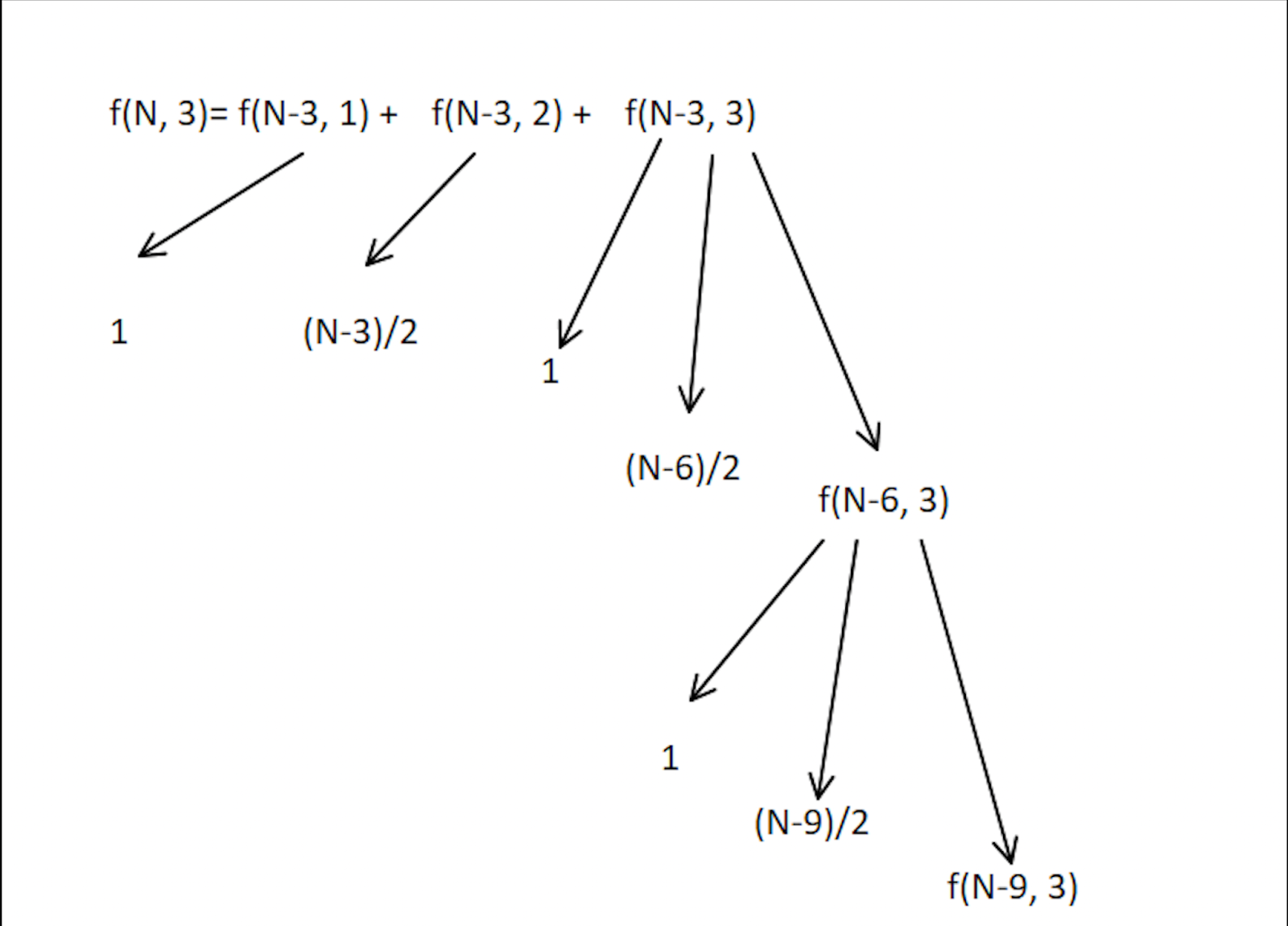# WEGMAC - Editorial

Author: Abhishek Ghosh
Tester: Rahul Sahay
Editorialist: Gaurav Kumar, Md. Sami Khan

Easy

# PREREQUISITES

Math, Distribution, DP, Precomputation

# PROBLEM

You have been given a weight of N kilos. The measure will be accurate only if 3 calibration weights are used. You need to put 3 weights such that the sum equals N kilos, given that the calibration weights are in multiples of K.

# QUICK EXPLANATION

• We have to divide N into 3 multiples of k (k{a}, k{b}, k{c}) such that:

k{a} + k{b} + k{c} = N \implies a+b+c = \frac{N}{k}

• The problem can be related to a common problem, distributing \frac{N}{k} identical balls into 3 identical boxes such that no box should be empty.

• Let f(N, m) be a function to distribute N identical balls into m identical boxes. In this particular problem:

f(N, 3)= f(N-3,1) + f(N-3, 2) + f(N-3, 3)

This recurrence relation is used to solve the problem.

# EXPLANATION

In the following problem, we have to split N into 3 multiples of k. None of the multiples should be 0.

If N is not divisible by K then the answer will simply be 0. Otherwise, we need to distribute \frac{N}{k} identical balls among 3 identical boxes, such that each should get at least one.

f(N, m) is a function to divide N into m multiples or to put N balls into m boxes such that no box is empty.

We give 1 ball to each of the 3 identical boxes and now we are left with N-3 balls to distribute without any restriction.

Now, we can either give all the N-3 balls to 1 box, or we can give the N-3 balls to 2 boxes, or we can give N-3 balls to all the 3 boxes which is nothing but f(N-3, 3), and similar to our initial condition that we started with.

Thus, f(N, 3) has 3 cases:

f(N, 3)= f(N-3,1)+f(N-3, 2)+f(N-3, 3)

Case 1

N-3 is given to just 1 box.

This can be done only in 1 way.

\implies f(N-3,1) = 1 for all N >= 3

Case 2

N-3 is given to just 2 boxes.

For N=3:
f(0,2)=0

For N=4:
f(1,2)=0

For N=5:
f(2,2)=1 {[1,1]}

For N=6:
f(3,2)=1 {[2,1]}

For N=7:
f(4,2)=2 {[3,1],[2,2]}

For N=8:
f(5,2)=2 {[4,1], [3,2]}

For N=9:
f(6,2)=3 {[5,1], [4,2], [3,3]}

For N=10:
f(7,2)=3 {[6,1], [5,2], [4,3]}

By recognizing the simple logic, or understanding the pattern we can say that this can be done in \frac{N-3}{2} ways.

\implies f(N-3,2)=\frac{N-3}{2} \; \forall N \geq 3

Case 3 (Actual case)

N-3 is given to all 3 multiples.
This can further be broken into 3 cases.
f(N-3, 3) = f(N-6,1) + f(N-6, 2) + f(N-6, 3)
This is the initial case that we started with.

f(N, 3)= 1 + \frac{N-3}{2} + f(N-3, 3)Approach 1:

We can call the recurrence relation for each value of N. But in this approach we will have to calculate the value of f(N-r, 3) multiple times, where 0<r<N. This approach is acceptable for smaller values of N and K.

Time complexity:
O(T\mathord{\cdot}N) where T is the number of test cases.

Approach 2:

We can use precomputation and store the final answer for each N in an array; the i^{th} index of the array gives the answer for N=i
Time complexity:
O(T + N) where T is the number of test cases.

# SOLUTIONS

Setter's Solution
#include <bits/stdc++.h>
#define mod 1000000007
using namespace std;

const int maxN = 1e6;
int dp[maxN+1];

int32_t main() {
#ifndef ONLINE_JUDGE
freopen("input.txt", "r", stdin);
freopen("output.txt", "w", stdout);
#endif
ios_base::sync_with_stdio(false);
cin.tie(NULL);
cout.tie(NULL);

// precompute till 10^6
dp = dp = dp = 0;
for(int i = 3; i <= maxN; ++i) {
dp[i] = (1 + (i-3)/2 + dp[i-3]) % mod;
}

int T = 1;
cin >> T;

while(T--) {
int n, k;
cin >> n >> k;
if(n%k) {
cout << 0 << "\n";
continue;
}
n = n/k;
cout << dp[n] << "\n";
}

return 0;
}